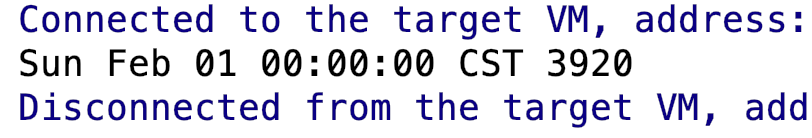# Java避坑之如何创建指定时间Date对象

``````Date date = new Date(2020, 1, 1);
System.out.println(date);
````````````@Deprecated
public Date(int year, int month, int date, int hrs, int min, int sec) {
int y = year + 1900;
// month is 0-based. So we have to normalize month to support Long.MAX_VALUE.
if (month >= 12) {
y += month / 12;
month %= 12;
} else if (month < 0) {
y += CalendarUtils.floorDivide(month, 12);
month = CalendarUtils.mod(month, 12);
}
BaseCalendar cal = getCalendarSystem(y);
cdate = (BaseCalendar.Date) cal.newCalendarDate(TimeZone.getDefaultRef());
cdate.setNormalizedDate(y, month + 1, date).setTimeOfDay(hrs, min, sec, 0);
getTimeImpl();
cdate = null;
}
``````

``````DateFormat df = new SimpleDateFormat("yyyy-MM-dd");
Date date = null;
try {
date = df.parse("2020-01-01");
} catch (ParseException e) {
e.printStackTrace();
}
System.out.println(date);
``````